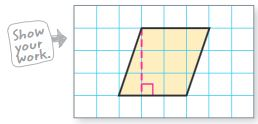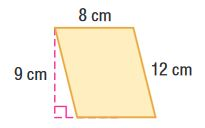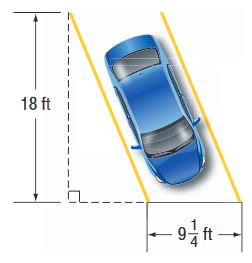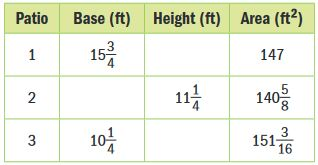Homework Explained - Math Practice 101Dear guest, you are not a registered member. As a guest, you only have read-only access to our books, tests and other practice materials.

As a registered member you can:

Registration is free and doesn't require any type of payment information. Click here to Register.
Go to page:
Chapter 9:Area;Lesson 1: Area of Parallelograms

Find the area of each parallelogram.

• Question 1•  $$\text{ units}^2.$$
• Question 2

base, 6 millimeters;
height, 4 millimeters

•  $$\text{ mm}^2.$$
• Question 3•  $$\text{ cm}^2.$$
• Question 4

Find the base of a parallelogram with an area of 24 square feet and height 3 feet.

•  feet
• Question 5

Find the area of the parking space shown to the right.•  sq. ft.
• Question 6

An architect designed three different parallelogram-shaped brick patios. Write the missing dimensions in the table.• Question 7

The base of a building is shaped like a parallelogram.The first floor has an area of 20,000 square feet. If the base of this parallelogram is 250 feet can its height be 70 feet? Explain.

• Question 8

Identify Structure Draw and label a parallelogram with a base twice as long as the height and an area less than 60 square inches. Find the area.

Yes, email page to my online tutor. (if you didn't add a tutor yet, you can add one here)